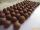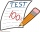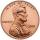# The King

The King wants to divide his sons equally. He has 42 rubies and 45 diamonds. How many sons and how will they share them?

Result

n =  3
r =  14
d =  15

#### Solution:Leave us a comment of example and its solution (i.e. if it is still somewhat unclear...):Be the first to comment!#### To solve this example are needed these knowledge from mathematics:

Do you want to calculate least common multiple two or more numbers? Do you have a linear equation or system of equations and looking for its solution? Or do you have quadratic equation?

## Next similar examples:

1. Balls groupsKarel pulled the balls out of his pocket and divide it into the groups. He could divide them in four, six or seven, and no ball ever left. How little could be a ball?
2. Counting numberWhat is the smallest counting number divisible by 2,5,7,8 and 15?
3. Examination 2In an examination, a teacher awards 2 marks for every correct answer and subtract a mark for every wrong answer. If there are 20 questions and a child scored 25 marks how many correct answer did the child get?
4. InfirmaryTwo thirds of children from the infirmary went on a trip seventh went to bathe and 40 children remained in the gym. How many children were treated in the infirmary?
5. Forest nurseryIn the forest nursery after winter, they found that 1/10 stems died out of them. For them, they land 193 new spruces. How many spruces are in the forest nursery?
6. Tickets 3A total of 645 tickets were sold for the school play. They were either adult tickets or student tickets. There were 55 fewer student tickets sold than adult tickets. How many adult tickets were sold?
7. Number with onesThe first digit of the number is 1, if we move this digit to the end we get a 3 times higher number, which is the number?
8. Last pageTwo consecutive sheets dropped out of the book. The sum of the numbers on the sides of the dropped sheets is 154. What is the number of the last page of the dropped sheets?
9. Dropped sheetsThree consecutive sheets dropped from the book. The sum of the numbers on the pages of the dropped sheets is 273. What number has the last page of the dropped sheets?
10. Find xSolve: if 2(x-1)=14, then x= (solve an equation with one unknown)
11. Negative in equation2x + 3 + 7x = – 24, what is the value of x?
12. Unknown number 11That number increased by three equals three times itself?
13. Simple equationSolve for x: 3(x + 2) = x - 18
14. Simple equationSolve the following simple equation: 2. (4x + 3) = 2-5. (1-x)
15. Cents no moreJanko bought pencils for 35 cents each. Neither he nor the salesperson had small coins just a whole € 1 coin. At least how many pencils had to buy to pay for the whole euros?
16. Lcm simpleFind least common multiple of this two numbers: 140 175.
17. Lcm of three numbersWhat is the Lcm of 120 15 and 5